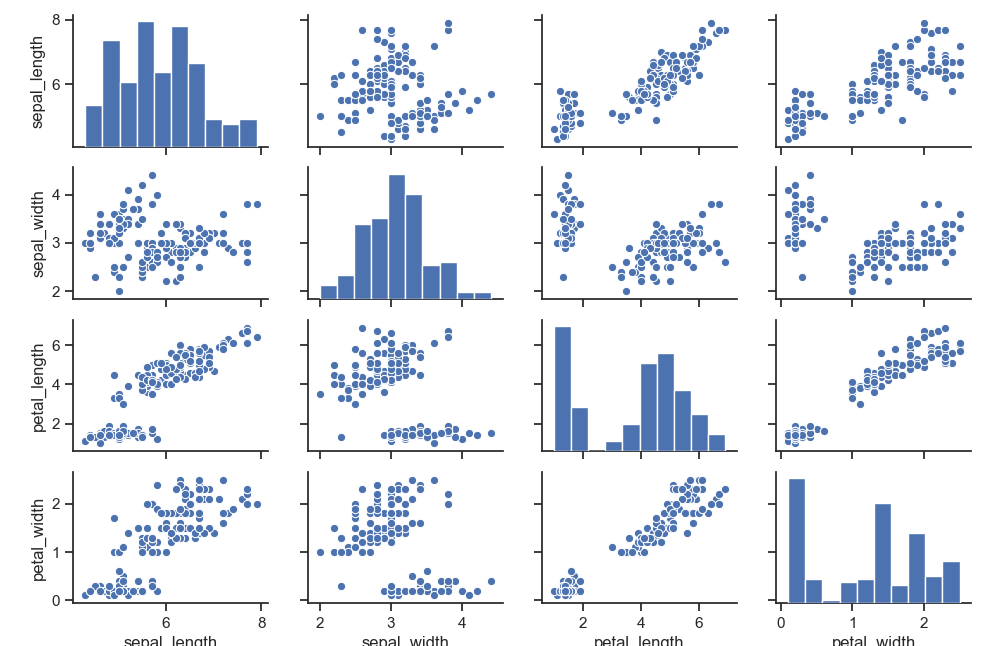The pairplot function creates a grid of Axes such that each variable in data will by shared in the y-axis across a single row and in the x-axis across a single column. That creates plots as shown below.

Related course: Matplotlib Examples and Video Course

## pairplot

### pairplot

The pairplot plot is shwon below. Its using the iris flower data set. The data set has 4 measurements: sepal width, sepal length, petal_length and petal_width. The data is mapped in the grid below. Because there are 4 measurements, it creates a 4x4 plot.If you prefer a smaller plot, use less variables. For instance, if you only want sepal_width and sepal_length, tha would create a 2x2 plot.

You can change the shape of the distribution.